# Bihar Board Class 10 Science Solutions Chapter 10 Light Reflection and Refraction

BSEB Bihar Board Class 10 Science Solutions Chapter 10 Light Reflection and Refraction Textbook Questions and Answers.

## Bihar Board Class 10 Science Solutions Chapter 10 Light Reflection and Refraction

### Bihar Board Class 10 Science Chapter 10 Light Reflection and Refraction InText Questions and Answers

In-text Questions (Page 168)

Question 1.
Define the principal focus of a concave mirror.
A point on the principal axis of a spherical mirror where the rays of light parallel to the principal axis meet after reflection from the spherical mirror is called the principal focus.

Question 2.
The radius of curvature of a spherical mirror is 20 cm. What is its focal length?
Focal Length f = $$\frac{\mathrm{R}}{2}$$
Here, R = 20 cm
therefore, f = 10 cm

Question 3.
Name a mirror that can give an erect and enlarged image of an object.
Concave mirror

Question 4.
Why do we prefer a convex mirror as a rearview mirror in vehicles?
A convex mirror always gives an erect though diminished image for an object placed at any distance from it. Moreover, it gives a wider field view so that the driver can see a lot more traffic behind him than that possible by a plane mirror. This enables him to drive safely.

In-text Questions (Page 171)

Question 1.
Find the focal length of a convex mirror whose radius of curvature is 32 cm.
Given, Radius of curvature, R = 32 cm
Focal length f = $$\frac{\mathrm{R}}{2}$$
∴ f = $$\frac{32}{2}$$ = 16 m
Hence, focal length is 16 m.

Question 2.
A concave mirror produces three times magnified (enlarged) real image of an object placed at 10 cm in front of it. Where is the image located?
Given, m = -3, u = -10 cm
Magnification, m = $$\frac{-v}{u}$$ ⇒ -3 = $$\frac{-v}{-10}$$
υ = -30 cm
Image is located 30 cm in front of mirror.

In-text Questions (Page 176)

Question 1.
A ray of light travelling in air enters obliquely into water. Does the light ray bend towards the normal or away from the normal? Why?
The ray of light will bend towards the normal as it is entering in denser medium (water) from rarer medium (air).

Question 2.
Light enters from air to glass having refractive index 1.50. What is the speed of light in the glass? The speed of light in vacuum is 3 × 108 ms-1.
Given, ng = 1.50, e = 3 × 108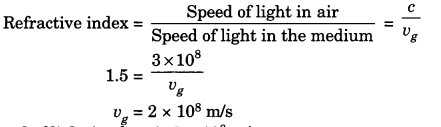Speed of light in glass is 2 × 108

Question 3.
Find out, from table 10.3 (NCERT Textbook page 175), the medium having higher optical density. Also find the medium with lowest optical density.
Minimum optical density: Air
Maximum optical density: Diamond

Question 4.
You are given kerosene, turpentine and water. In which of these does the light travels fastest? Use the information given in table 10.3.
Light travels fastest in a medium having minimum optical density or refractive index. Since water has the least refractive index, the speed of light is fastest in water.

Question 5.
The refractive index of diamond is 2.42. What is the meaning of this statement?
According to this statement light travels 2.42 times slower in diamond as compared to vacuum or air.

In-text Questions (Page 184)

Question 1.
Define 1 dioptre of power of a lens.
1 dioptre is the power of a lens whose focal length is 1 metre.

Question 2.
A convex lens forms a real and inverted image of a needle at a distance of 50 cm from it. Where is the needle placed in front of the convex lens if the image is equal to the size of the object? Also find out the power of the lens.
Here υ = 50 cm, m = -1
Magnification m = $$\frac{v}{u}$$ ⇒ -1 = $$\frac{(50)}{u}$$
∴ u = -50 cm
Object is placed 50 cm in front of the mirror.
For power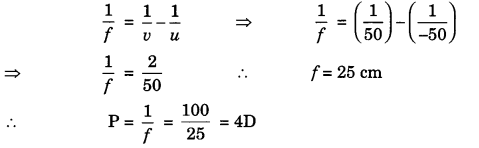Question 3.
Find the power of concave lens of focal length 2 m.
Here, f= 2 m
p = $$\frac{1}{f}$$ ⇒ P = $$\frac {1}{2}$$ = 0.5D
Power of lens is 0.5D.

### Bihar Board Class 10 Science Chapter 10 Light Reflection and Refraction Textbook Questions and Answers

Question 1.
Which one of the following materials cannot be used to make a lens?
(a) Water
(b) Glass
(c) Plastic
(d) Clay
(d) Clay cannot be used to make a lens as it is not transparent.

Question 2.
The image formed by a concave mirror is observed to be virtual, erect and larger than the object. Where should be the position of the object?
(a) Between the principal focus and the centre of curvature
(b) At the centre of curvature
(c) Beyond the centre of curvature
(d) Between the pole of the mirror and its principal focus.
(d) Between the pole of the mirror and its principal focus.

Question 3.
Where should an object be placed in front of a convex lens to get a real image of the size of the object?
(а) At the principal focus of the lens
(b) At twice the focal length
(c) At infinity
(d) Between the optical centre of the lens and its principal focus.
(b) At twice the focal length

Question 4.
A spherical mirror and a thin spherical lens have each a focal length of -15 cm. The mirror and the lens are likely to be
(a) both concave.
(b) both convex.
(c) the mirror is concave and the lens is convex.
(d) the mirror is convex, but the lens is concave.
(a) both concave.

Question 5.
No matter how far you stand from a mirror, your image appears erect. The mirror is likely to be
(a) plane
(b) concave
(c) convex
(d) either plane or convex.
(d) either plane or convex.

Question 6.
Which of the following lenses would you prefer to use while reading small letters found in a dictionary?
(a) A convex lens of focal length 50 cm.
(b) A concave lens of focal length 50 cm.
(c) A convex lens of focal length 5 cm.
(d) A concave lens of focal length 5 cm.
(a) A convex lens of focal length 5 cm.

Question 7.
We wish to obtain an erect image of an object, using a concave mirror of focal length 15 cm. What should be the range of distance of the object from the mirror? What is the nature of the image? Is the image larger or smaller than the object? Draw a ray diagram to show the image formation in this case.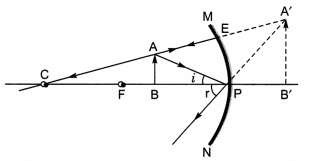Object should be placed between pole and principal focus of the mirror. Range of distance is less than 15 cm. The image will be virtual, erect, large sized and behind the mirror.

Question 8.
Name the type of mirror used in the following situations:
(b) Side/rear-view mirror of a vehicle
(c) Solar furnace.
(a) Concave mirror: for getting powerful beam of light.
(b) Convex mirror: for getting erect and small sized image.
(c) Concave mirror: for concentrating sunlight to produce heat.

Question 9.
One-half of a convex lens is covered with a black paper. Will this lens produce a complete image of the object? Verify your answer experimentally. Explain your observations.
Yes, it will produce a complete image of the object. But the image will be less bright as some amount of light will be blocked by the black paper.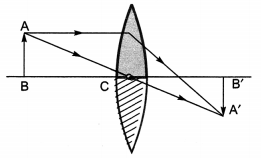Question 10.
An object 5 cm in length is held 25 cm away from a converging lens of focal length 10 cm. Draw the ray diagram and find the position, size and the nature of the image formed.
u = -25 cm, f = 10 cm, h = 5 cm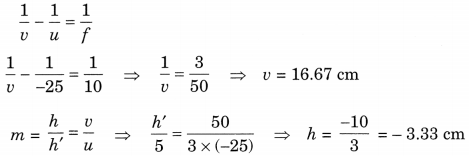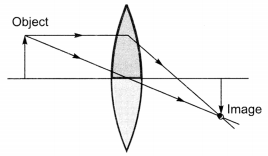Image is real and inverted.

Question 11.
A concave lens of focal length 15 cm forms an image 10 cm from the lens. How far is the object placed from the lens? Draw the ray diagram.
Here, υ = -10 cm, f = 15 cm, u = ?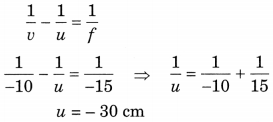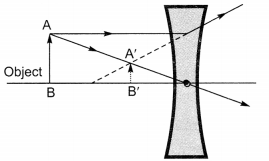Question 12.
An object is placed at a distance of 10 cm from a convex mirror of focal length 15 cm. Find the position and nature of the image.
Here, u = -10 cm, f = 15 cm, υ = ?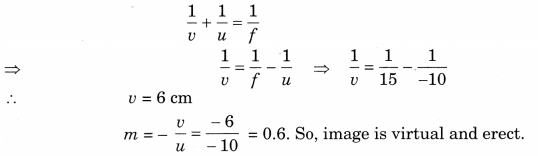Question 13.
The magnification produced by a plane mirror is +1. What does this mean?
m = $$\frac{h^{\prime}}{h}$$ = 1
h’ = h
Image produced by plane mirror is same sized, virtual and erect.

Question 14.
An object 5.0 cm in length is placed at a distance of 20 cm in front of a convex mirror of radius of curvature 30 cm. Find the position of the image, its nature and size.
Height of object (h) = 5 cm
u = -20 cm; f = +15 cm, R = +30 cm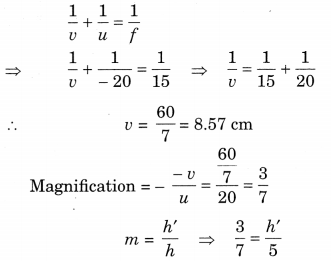∴ h’ = $$\frac{15}{7}$$ = 2.14 cm
Since h’ is positive, and m < 1 image is virtual, erect and diminished.

Question 15.
An object of size 7.0 cm is placed at 27 cm in front of a concave mirror of focal length 18 cm. At what distance from the mirror should a screen be placed, so that a sharp focussed image can be obtained? Find the size and the nature of the image.
Size of object (h) = 7 cm, u = -21 cm, f = -18 cm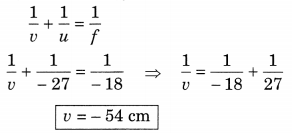At a distance of 54 cm sharp image can be obtained.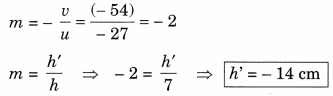Image is real, inverted and enlarged.

Question 16.
Find the focal length of a lens of power – 2.0 D. What type of lens is this?
f = $$\frac{1}{\mathrm{P}}$$, f = $$\frac{1}{-2}$$ = 0.5 cm, f = -50 cm
Focal length is negative, lens is concave.

Question 17.
A doctor has prescribed a corrective lens of power + 1.5 D. Find the focal length of the lens. Is the prescribed lens diverging or converging?
f = $$\frac{1}{\mathrm{P}}$$, f = $$\frac{1}{1.5}$$ = = 0.67 m = + 67 cm
Focal length is positive, lens is converging.

### Bihar Board Class 10 Science Chapter 10 Light Reflection and Refraction Textbook Activities

Activity 10.1 (Textbook page 161)

Discussion and Conclusion:
The inner curved surface of a large shining spoon acts as a concave mirror. Our face is the object. When the spoon is at a small distance from the mirror, the object lies between pole P and focus F of concave mirror. We observe enlarged, erect image of our face behind the spoon. When we move the spoon slowly away from our face, we observe that

• When face is at focus of mirror/spoon, highly enlarged, inverted image of our face is seen at infinity.
• When face is between F and C of mirror/spoon, an enlarged, inverted image of our faces is seen.
• When face is at the centre of curvature of the mirror/spoon, an inverted image of same size is seen.
• When face is beyond C, an inverted and diminished image of our face is seen.
• When the face is very far off, highly diminished, inverted image of our face is seen at the focus of spoon/mirror.

When we reverse the spoon, its curved portion is bulged out. It behaves as a convex mirror. The image of our face is virtual, erect and diminished. As we move the spoon away from our face, image moves away till it is at the focus of the mirror. The image, however, continues to be virtual, erect and finally, it is diminished to almost point size.

Activity 10.2 (Textbook page 162)

Discussion and Conclusion:
The source of light, the sun in this case is at infinite distance from the concave mirror. Therefore, on reflection from the concave mirror, the sunlight collects at the focus of the mirror. By moving the sheet of paper back and forth gradually, we find a bright, sharp spot of light on the paper. This spot is the real, point size image of the Sun at the focus of the concave mirror (see figure).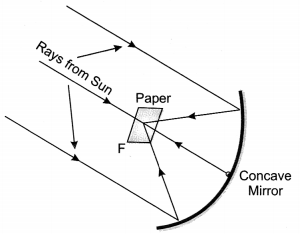When we hold the mirror and the paper in the same position for a few minutes, the paper may burn out. This is because the Sun rays are concentrated on the bright, sharp spot of light on the paper.

Activity 10.5 (Textbook page 167)

Discussion and Conclusion:
When we hold a pencil in the upright position in front of a convex mirror, we observe the image of the pencil in the mirror, at the back of the mirror. The image is erect, virtual and smaller in size than the object.

As the pencil is moved away from the mirror, the image becomes smaller and smaller, moving away from the mirror.

On repeating the activity, we find that as the object is moved away from the mirror, the image would move closer to the focus of the mirror.

Activity 10.6 (Textbook page 167)

Discussion and Conclusion:
When we observe the image of a distant object, say a distant tree, in a plane mirror, we may not see a full length image. When we try with plane mirrors of different sizes, we find that the image of the entire object is seen when size of plane mirror is atleast half the size of the object.

When we repeat this activity with a concave mirror, we do see full length image of the object for certain locations of the object.

Again, when we use a convex mirror, the full length image of an object is seen, wherever the object may be located. The reasons behind these observations are that

• in a plane mirror, size of image is always equal to the size of the object,
• in a concave mirror, the image is equal in size or smaller in size to the size of the object only for certain locations of the object, and
• in a convex mirror, the image is always virtual, erect and shorter than the object, wherever the object maybe located.

Activity 10.7 (Textbook page 172)

Discussion and Conclusion:
Figure shows a coin O at the bottom of a bucket filled with water. When we view this coin with our eye to a side above water, we observe the image I of the coin, which is above O. When we try to pick up this coin in one go, we do not succeed in picking up the coin. This is because we move our hand upto I, where the coin is being observed, but actually the coin lies below at O, the bottom of the bucket. When our friends try the same way, they also fail to pick up the coin. However, if some one understands that the coin is at the bottom of the bucket, he/she can pick up the coin.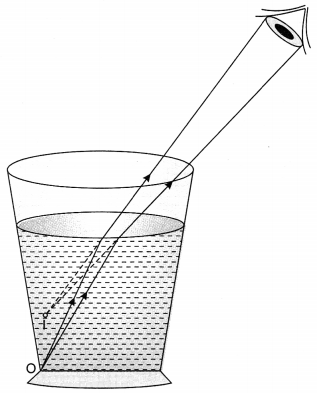Activity 10.8 (Textbook page 172)

Discussion and Conclusion:
This activity is an extension of the last activity 10.7. In a large shallow bowl filled with water, the coin is at the bottom at O. On account of refraction of light, image I of the coin appears to be raised. When we move our eye slowly away from the bowl, the coin disappears from our sight. This happens when rays starting from coin O fail to enter our eye, after refraction at the water surface.

When a friend pours more water gently into the bowl without disturbing the coin, the coin becomes visible again from the same position of our eye. This happens because on adding water, real depth of the coin increases. The apparent depth of the coin is equal to real depth divided by refractive index of water. Therefore, apparent depth of the coin also increases. The apparent position of the coin rises slightly above I and it becomes visible from the same position of our eye.

Activity 10.9 (Textbook page 172)

Discussion and Conclusion:
Figure shows a thick straight line ABCD in ink drawn over a sheet of white paper. A glass slab PQRS is placed over the line such that edge PQ of the slab makes an acute angle with the line ABCD.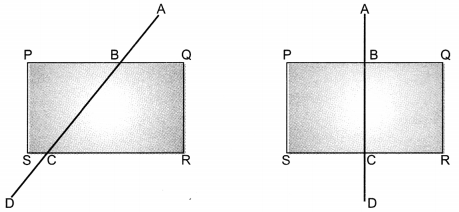When we look at the portion BC of the line under the slab, from the sides, careful observation shows that the line is bent at the edges B and C. This is because of refraction of light. At B, light enters from air to glass. Therefore, it bends towards the normal. At C, light enters from glass to air. Therefore, it bends away from the normal. The emergent ray CD, after two refractions, is parallel to AB, but is slightly displaced laterally. When the glass slab is so placed that one of its edges PQ is normal to the line ABCD, the part BC of the line under the slab does not appear to be bent. This is because for the incident ray AB, angle of incidence i = 0. Therefore, angle of refraction r is also zero. Therefore, no refraction occurs at B, and there is no bending. Similarly, no refraction occurs at C and hence there is no bending at C.

When we look at the portion of the line BC from the top of the glass slab, it appears to be raised. This is also an account of refraction of light. Rays of light starting from BC pass from glass to air. When viewed from the top of slab, these rays bend away from the normal. Therefore, the image of portion BC of the line appears to be raised.

Activity 10.11 (Textbook page 177)

Discussion and Conclusion:
When we direct a convex lens in our hand towards the Sun, the light from the Sun is focussed on a sheet of paper. By moving the sheet of paper back and forth, we obtain a sharp, bright image of the Sun on the paper.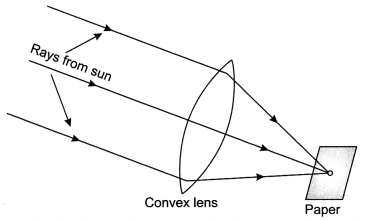When the paper and lens are held in the same position for the some time, the paper may burn out. This is because of heat produced by the Sun rays concentrate on the spot where the image of Sun is formed. This is what we observed in Activity 10.2.